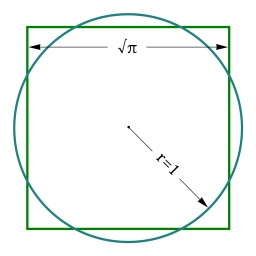# Circumference 6338

The circle has the same area as the square, whose circumference is 400m. Calculate the diameter of the circle.

D =  112.8379 m

### Step-by-step explanation:Did you find an error or inaccuracy? Feel free to write us. Thank you!

Tips for related online calculators
Do you have a linear equation or system of equations and looking for its solution? Or do you have a quadratic equation?

#### Grade of the word problem:

We encourage you to watch this tutorial video on this math problem: